# Perron-Stieltjes integral

(diff) ← Older revision | Latest revision (diff) | Newer revision → (diff)

A generalization of the Perron integral for a function of one real variable. A finite functionis said to be integrable in the sense of Perron–Stieltjes with respect to a finite functiononif onthere exists a major functionand a minor functionforwith respect toonhavingand such that at each point,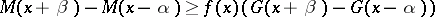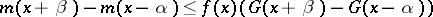for all sufficiently small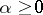and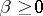, while the greatest lower bound of the numbers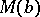, whereis any such major function ofwith respect to, and the least upper bound of the numbers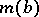, whereis any such minor function ofwith respect to, coincide. Their common value is called the Perron–Stieltjes integral ofwith respect toonand is denoted by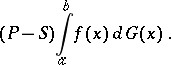This generalization of the Perron integral was introduced by A.J. Ward .

How to Cite This Entry:
Perron-Stieltjes integral. Encyclopedia of Mathematics. URL: http://encyclopediaofmath.org/index.php?title=Perron-Stieltjes_integral&oldid=12939
This article was adapted from an original article by T.P. Lukashenko (originator), which appeared in Encyclopedia of Mathematics - ISBN 1402006098. See original article